• 3-7 表达式转换 (20分) 算术表达式有前缀表示法、中缀表示法和后缀表示法等形式。日常使用的算术表达式是采用中缀表示法，即二元运算符位于两个运算数中间。请设计程序将中缀表达式转换为后缀表达式。 输入格式: ...
3-7 表达式转换 (20分)
算术表达式有前缀表示法、中缀表示法和后缀表示法等形式。日常使用的算术表达式是采用中缀表示法，即二元运算符位于两个运算数中间。请设计程序将中缀表达式转换为后缀表达式。
输入格式:
输入在一行中给出不含空格的中缀表达式，可包含+、-、*、\以及左右括号()，表达式不超过20个字符。
输出格式:
在一行中输出转换后的后缀表达式，要求不同对象（运算数、运算符号）之间以空格分隔，但结尾不得有多余空格。
输入样例:

2+3*(7-4)+8/4

输出样例:

2 3 7 4 - * + 8 4 / +

代码
#include<iostream>
#include<algorithm>
#include<string>
#include<stack>
using namespace std;
bool isNum(char c){
if(c>='0'&&c<='9') return true;
else return false;
}
int main(){
ios::sync_with_stdio(false);
cin.tie(0),cin.tie(0);
string s,res;
cin>>s;
int len=0;
for(int i=0;i<s.length();i++){
if(isNum(s[i])||s[i]=='.') len++;
}
if(len==s.length()){
cout<<s;
return 0;
}
if(s=='-') {s=s.substr(1);cout<<"-";}
stack<char> st;
for(int i=0;i<s.length();i++){
if(s=='-'||isNum(s[i])){
int j=i+1;
while(isNum(s[j])||s[j]=='.'){
j++;
}
res+=s.substr(i,j-i);
res+=' ';
i=j-1;
}else{
if(s[i]=='+'&&s[i-1]!='('||s[i]=='-'&&s[i-1]!='('){
while(!st.empty()&&st.top()!='('){
res+=st.top();res+=" ";
st.pop();
}
st.push(s[i]);
}else if(s[i]=='-'&&s[i-1]=='('){
int j=i+1;
while(isNum(s[j])||s[j]=='.'){
j++;
}
res+=s.substr(i,j-i);
res+=' ';
i=j-1;
}else if(s[i]=='*'||s[i]=='/'||s[i]=='('){
st.push(s[i]);
}else if(s[i]==')'){
while(st.top()!='('){
res+=st.top();
res+=" ";
st.pop();
}
st.pop();
}
}
}
while(!st.empty()){
res+=st.top();
if(st.size()>1)
res+=" ";
st.pop();
}
cout<<res;
return 0;
}



展开全文• 表达式转换 算术表达式有前缀表示法、中缀表示法和后缀表示法等形式。日常使用的算术表达式是采用中缀表示法，即二元运算符位于两个运算数中间。请设计程序将中缀表达式转换为后缀表达式。 输入格式: 输入在一行中...
表达式转换
算术表达式有前缀表示法、中缀表示法和后缀表示法等形式。日常使用的算术表达式是采用中缀表示法，即二元运算符位于两个运算数中间。请设计程序将中缀表达式转换为后缀表达式。
输入格式:
输入在一行中给出不含空格的中缀表达式，可包含+、-、*、\以及左右括号()，表达式不超过20个字符。
输出格式:
在一行中输出转换后的后缀表达式，要求不同对象（运算数、运算符号）之间以空格分隔，但结尾不得有多余空格。
输入样例:
2+3*(7-4)+8/4
输出样例:
2 3 7 4 - * + 8 4 / +
知识点：将中缀表达式转化为后缀表达式：
两种情况：
（1）没有括号的中缀表达式转化为后缀表达式：（2）带有括号的中缀表达式转化为后缀表达式：分析：本题是带有括号的中缀表达式，需要利用第二种方式实现，模拟过程我会在代码上详解
这里说一下这道题的细节问题：
中缀表达式转换为后缀表达式：对于本题而言，细节过多，在完成表达式转换的基础上
1.需要控制空格，首尾不能出现空格
2.需要判断一位以上的数字之间不能有空格
3.需要判断小数的情况，注意之间不能有空格
4.需要判断镶嵌括号的情况，容易出现格式错误
例如：当一开始就输入多个空格会使首位出现空格
5.需要判断正负号
（1）对于正号，当出现在第一位时需直接特判（在第一位无需加括号）
未在第一位出现正号一定会在括号内，而且输出时正号要省略
（2）对于负号，当出现在第一位时需要直接特判（在第一位出现无需加括号）
未在第一位出现负号一定会在括号内，需要输出负号
（3）注意控制好空格，防止格式错误
下面是代码：

#include<cstdio>
#include<cstring>
#include<iostream>
#include<stack>
#include<algorithm>
using namespace std;
const int M=1010;
char str[M],st[M],c;
stack<char> q;
int main()
{
int i,j,k=0,l,m,n,f=0,t=0,ff=0;
cin.getline(str,110);
l=strlen(str);
for(i=0; i<l; i++)
{
if(str[i]>='0'&&str[i]<='9')///为数字时输出
{
t=1;///标记已经出现过数字
///解决当有镶嵌括号时的格式问题
if(ff==0)///特判当刚开始有镶嵌括号时防止f=1对格式的影响
{
printf("%c",str[i]);
ff=1;
f=0;
continue;
}
///解决超过一位数的问题
if(f==0)///遇到数字并且前一个也是数字或'.'输出
printf("%c",str[i]);
else ///f=1时遇到数字加空格输出，并使f=0
{
printf(" %c",str[i]);
f=0;
}
}
///解决小数问题
else if(str[i]=='.')///遇到点说明为小数直接输出
{
printf("%c",str[i]);
}
///解决第一位为正数带'+'的问题
else if(i==0&&str[i]=='+')
{
printf("%c",str[i]);
}
///解决第一位为正数带'-'的问题
else if(i==0&&str[i]=='-')
{
printf("%c",str[i]);
}
///解决非第一位为正数带'-'的问题
else if(str[i]=='('&&str[i+1]=='-')
{
///输出符号
if(t==1)///若已经出现数字，加符号时需在前面加空格
printf(" %c",str[i+1]);
else
printf("%c",str[i+1]);
q.push(str[i]);///将括号入栈
f=0;///输出数字无需加空格
i++;///跳过这个'-'
}
///解决非第一位为正数带'+'的问题
else if(str[i]=='('&&str[i+1]=='+')
{
///带正号的数字的正号可以约去
q.push(str[i]);///将括号入栈
f=1;///输入数字时需要加空格
i++;///跳过'+'
}
///当遇见左括号
else if(str[i]=='(')
{
q.push(str[i]);///放入栈中
f=1;
}
///遇见右括号
else if(str[i]==')')
{
///输出左括号到右括号之间的符号
while(q.top()!='(')
{
printf(" %c",q.top());
f=1;///当输入数字时需加空格
q.pop();///将输出的字符出栈
}
//printf("%c\n",q.top());
q.pop();///将左括号出栈
}
else
{
///当栈为空或者栈顶元素为左括号
if(q.size()==0||q.top()=='(')
{
f=1;///输入数字时需要加空格
q.push(str[i]);///将符号放入栈中
}
///当需要入栈的符号优先级大于栈顶符号优先级
else if((str[i]=='*'||str[i]=='/')&&(q.top()=='+'||q.top()=='-'))
{
f=1;///输入数字时需加括号
q.push(str[i]);///将符号入栈
}
///入栈的符号优先级小于或等于栈顶符号优先级
///栈中元素不为空且栈顶元素不是左括号
else
{
///当栈顶元素为'*'或'/'
if(q.top()=='*'||q.top()=='/')
{
f=1;///输入数字需加空格
printf(" %c",q.top());///加空格输出栈顶元素
q.pop();///将栈顶元素出栈
///出栈完看栈中元素是否为空

///此时四种情况
///（1）栈中元素为0
///（2）栈顶元素为'('
///（3）栈底元素为'+'或'-'，输入符号为'*'或'/'
///（4）栈底元素为'+'或'-'，输入符号为'+'或'-'

///情况一和情况二
if(q.size()==0||q.top()=='(')
{
f=1;///输出数字时需加空格
q.push(str[i]);///将符号放入栈中
}
///情况三
else if(str[i]=='*'||str[i]=='/')
{
f=1;///输出数字时需加空格
q.push(str[i]);///直接将符号入栈
}
///情况四
else if(str[i]=='+'||str[i]=='-')
{
f=1;///输出数字时需加空格
printf(" %c",q.top());///将栈顶元素加空格输出
q.pop();///栈顶元素出栈
q.push(str[i]);///将符号放入栈中
}
}
///当栈顶元素为'+'或'-'
else if(q.top()=='+'||q.top()=='-')
{
///两种情况
///（1）入栈符号为'*'或'/'
///（2）入栈符号为'+'或'-'

///情况一
if(str[i]=='*'||str[i]=='/')
{
f=1;///输出数字时需加空格
q.push(str[i]);///将符号放入栈中
}
else if(str[i]=='+'||str[i]=='-')
{
f=1;///输出数字时需加空格
printf(" %c",q.top());///输出栈顶元素
q.pop();///栈顶元素出栈
q.push(str[i]);///将符号放入栈中
}
}
}
}
}
///当栈中符号未完全出栈
while(q.size()!=0)
{
printf(" %c",q.top());///将栈中符号依次输出
q.pop();///依次出栈
}
printf("\n");
return 0;
}




展开全文• 中缀表达式转换为后缀表达式 思路分析 代码实现 package com.atguigu.stack; import javax.swing.plaf.nimbus.State; import java.security.AlgorithmConstraints; import java.util.ArrayList; import java.util....
中缀表达式转换为后缀表达式
思路分析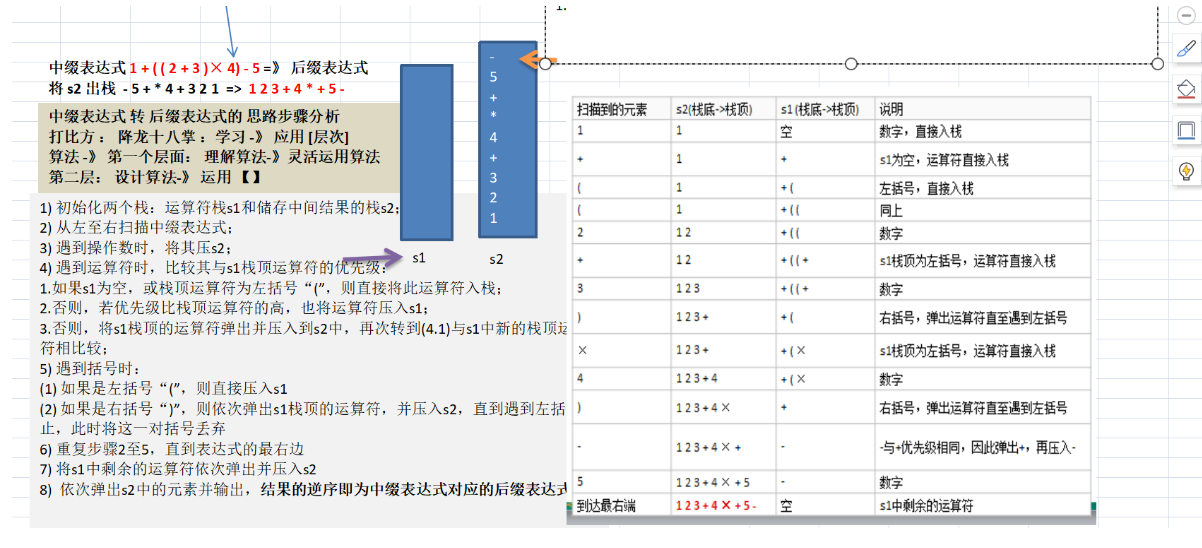代码实现
package com.atguigu.stack;

import javax.swing.plaf.nimbus.State;
import java.security.AlgorithmConstraints;
import java.util.ArrayList;
import java.util.List;
import java.util.Stack;

/**
* @创建人 wdl
* @创建时间 2021/3/20
* @描述
*/
public class PolandNotation {
public static void main(String[] args) {
//完成一个中缀表达式转换成后缀表达式的功能
//说明
//1."1+((2+3)×4)-5"=>"1 2 3 + 4 × + 5 –"
//2.因为直接对一个str进行操作，不方便，因此先将"1+((2+3)×4)-5"=>中缀的表达式对应的List
//即"1+((2+3)×4)-5"=>ArrayList[....
//3.将得到的中缀表到时对应的List转换成后缀表达式List

String expression="1+((2+3)*4)-5";
List<String> infixExpressionList = toInfixExpressionList(expression);
System.out.println("中缀表达式对应的List"+infixExpressionList);
List<String> parseSuffixExpreesionList = parseSuffixExpreesionList(infixExpressionList);
System.out.println("后缀表达式对应的List"+parseSuffixExpreesionList);

System.out.println("expression="+calculate(parseSuffixExpreesionList));

//        //先定义一个逆波兰表达式
//        // (3+4)×5-6 对应的后缀表达式就是 3 4 + 5 × 6 -
//        //说明为了方便，逆波兰表达式的数字和符号使用空格隔开
//        String suffixExpression="3 4 + 5 * 6 -";
//        //思路
//        //1.先将"3 4 + 5 × 6 -"放到ArrayList中
//        //2.将ArrayList传递给一个方法，遍历ArrayList配合栈完成计算
//        List<String> rpnList=getListString (suffixExpression);
//        System.out.println(rpnList);
//
//        int res=calculate(rpnList);
//        System.out.println("计算的结果是="+res);

}

public static List<String> parseSuffixExpreesionList(List<String> ls){
//定义两个栈
Stack<String> s1=new Stack<String>();//符号栈
//说明：因为s2这个栈，在整个转换过程中，没有pop操作，而且我们后面还需要逆序输出
//因此比较麻烦，这里我们就不用Stack<String>直接使用List<String> s2
//Stack<String> s2=new Stack<String>();存储中间结果的栈s2
List<String> s2 = new ArrayList<>();//存储中间结果的List2

//遍历ls
for(String item:ls){
//如果是一个数，加入到s2
if (item.matches("\\d+")){
}else if (item.equals("(")){
s1.push(item);
}else if(item.equals(")")){//如果是右括号“)”，则依次弹出s1栈顶的运算符，并压入s2，
// 直到遇到左括号为止，此时将这一对括号丢弃
while (!s1.peek().equals("(")){
}
s1.pop();//！！！将（弹出s1栈，消除小括号
}else{
//当item的优先级小于等于栈顶运算符的优先级，将s1栈顶的运算符弹出并加入到s2中，
// 再次转到(4.1)与s1中新的栈顶运算符相比较；
//问题：我们缺少一个比较优先级高低的方法
while (s1.size()!=0&&Operation.getValue(s1.peek()) >= Operation.getValue(item)){
}
//还需要将item压入栈
s1.push(item);
}

}

//将s1中剩余的运算符依次弹出并加入s2
while (s1.size()!=0){
}
return s2;//注意因为是存放到List，因此按顺序输出就是对应的后缀表达式对应的List

}

//方法：将中缀表达式转换成对应的List
public static List<String> toInfixExpressionList(String s){
//定义一个List，存放中缀表达式对应的内容
List<String> ls = new ArrayList<>();
int i=0;//这时是一个指针，用于遍历中缀表达式字符串
String str;//对多位数的拼接工作
char c;//每遍历一个字符，就放入到c
do{
//如果c是一个非数字，我们就需要加入到ls
if((c=s.charAt(i))<48||(c=s.charAt(i))>57){
i++;//i需要后移
}else {//如果是一个数，需要考虑多位数
str="";//先将str置成""
while (i<s.length()&&((c=s.charAt(i))>=48&&(c=s.charAt(i))<=57)){
str+=c;//拼接
i++;
}
}

}while (i<s.length());
return ls;//返回

}

//将一个逆波兰表达式，依次将数据和运算符放到ArrayList中
public static List<String> getListString(String suffixExpression){
//将suffixExpression分割
String[] split = suffixExpression.split(" ");
List<String> list = new ArrayList<>();
for(String ele:split){
}
return list;
}

//完成对逆波兰表达式的运算
//    从左至右扫描，将3和4压入堆栈；
//    遇到+运算符，因此弹出4和3（4为栈顶元素，3为次顶元素），计算出3+4的值，得7，再将7入栈；
//    将5入栈；
//    接下来是×运算符，因此弹出5和7，计算出7×5=35，将35入栈；
//    将6入栈；
//    最后是-运算符，计算出35-6的值，即29，由此得出最终结果
public static int calculate(List<String> ls){
//创建一个栈，只需要一个栈即可
Stack<String> stack = new Stack<>();
//遍历 ls
for(String item:ls){
//这里使用正则表达式来取出数
if(item.matches("\\d+")){//匹配的是多位数
//入栈
stack.push(item);
}else {
//pop出两个数，并运算，再入栈
int num2=Integer.parseInt(stack.pop());
int num1=Integer.parseInt(stack.pop());
int res=0;
if(item.equals("+")){
res=num1+num2;
}else if(item.equals("-")){
res=num1-num2;
}else if(item.equals("*")){
res=num1*num2;
}else if(item.equals("/")){
res=num1/num2;
}else {
throw new RuntimeException("运算符有误");
}
//把res入栈
stack.push(res+"");
}
}
//最后留在stack中的数据就是运算结果
return Integer.parseInt(stack.pop());

}

}

//编写一个类Operation返回一个运算符对应的优先级
class Operation{
private static int SUB=1;
private static int MUL=2;
private static int DIV=2;
//写一个方法，返回对应的优先级数字
public static int getValue(String operation){
int result=0;
switch (operation){
case "+":
break;
case "-":
result=SUB;
break;
case "*":
result=MUL;
break;
case "/":
result=DIV;
break;
default:
System.out.println("不存在该运算符");
break;
}
return result;
}

}



展开全文字符串 java 数据结构 stack
• ## 中缀表达式转换为后缀表达式

万次阅读 多人点赞 2017-04-22 11:50:10
一、后缀表达式求值 后缀表达式也叫逆波兰表达式，其求值过程可以用到栈来辅助存储。假定待求值的后缀表达式为：6 5 2 3 + 8 * + 3 + *，则其求值过程如下： 1）遍历表达式，遇到的数字首先放入栈中，此时栈如下所...
一、后缀表达式求值

后缀表达式也叫逆波兰表达式，其求值过程可以用到栈来辅助存储。假定待求值的后缀表达式为：6  5  2  3  + 8 * + 3  +  *，则其求值过程如下：

1）遍历表达式，遇到的数字首先放入栈中，此时栈如下所示：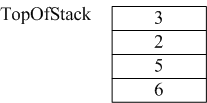2）接着读到“+”，则弹出3和2，执行3+2，计算结果等于5，并将5压入到栈中。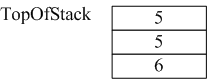3）读到8，将其直接放入栈中。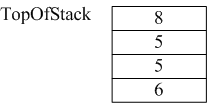4）读到“*”，弹出8和5，执行8*5，并将结果40压入栈中。而后过程类似，读到“+”，将40和5弹出，将40+5的结果45压入栈...以此类推。最后求的值288。

二、中缀表达式转后缀表达式

2.1）规则

中缀表达式a + b*c + (d * e + f) * g，其转换成后缀表达式则为a b c * + d e * f  + g * +。

转换过程需要用到栈，具体过程如下：

1）如果遇到操作数，我们就直接将其输出。

2）如果遇到操作符，则我们将其放入到栈中，遇到左括号时我们也将其放入栈中。

3）如果遇到一个右括号，则将栈元素弹出，将弹出的操作符输出直到遇到左括号为止。注意，左括号只弹出并不输出。

4）如果遇到任何其他的操作符，如（“+”， “*”，“（”）等，从栈中弹出元素直到遇到发现更低优先级的元素(或者栈为空)为止。弹出完这些元素后，才将遇到的操作符压入到栈中。有一点需要注意，只有在遇到" ) "的情况下我们才弹出" ( "，其他情况我们都不会弹出" ( "。

5）如果我们读到了输入的末尾，则将栈中所有元素依次弹出。

2.2）实例

规则很多，还是用实例比较容易说清楚整个过程。以上面的转换为例，输入为a + b * c + (d * e + f)*g，处理过程如下：

1）首先读到a，直接输出。

2）读到“+”，将其放入到栈中。

3）读到b，直接输出。

此时栈和输出的情况如下：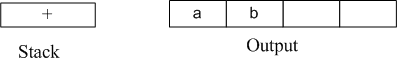4）读到“*”，因为栈顶元素"+"优先级比" * " 低，所以将" * "直接压入栈中。

5）读到c，直接输出。

此时栈和输出情况如下：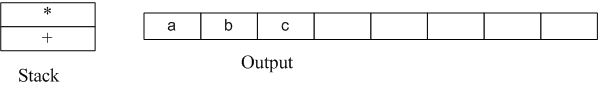6）读到" + "，因为栈顶元素" * "的优先级比它高，所以弹出" * "并输出， 同理，栈中下一个元素" + "优先级与读到的操作符" + "一样，所以也要弹出并输出。然后再将读到的" + "压入栈中。

此时栈和输出情况如下：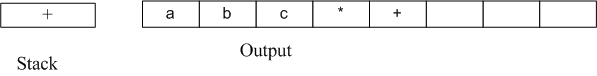7）下一个读到的为"("，它优先级最高，所以直接放入到栈中。

8）读到d，将其直接输出。

此时栈和输出情况如下：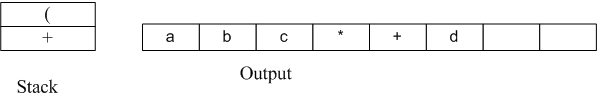9）读到" * "，由于只有遇到" ) "的时候左括号"("才会弹出，所以" * "直接压入栈中。

10）读到e，直接输出。

此时栈和输出情况如下：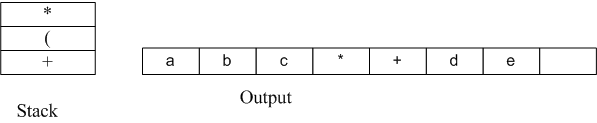11）读到" + "，弹出" * "并输出，然后将"+"压入栈中。

12）读到f，直接输出。

此时栈和输出情况：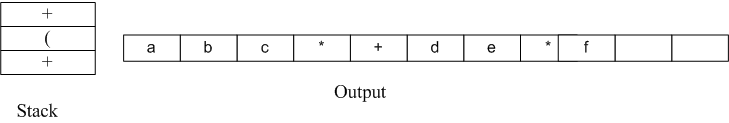13）接下来读到“）”，则直接将栈中元素弹出并输出直到遇到"("为止。这里右括号前只有一个操作符"+"被弹出并输出。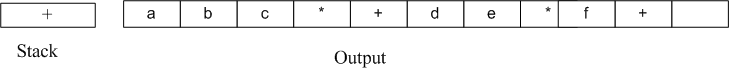14）读到" * "，压入栈中。读到g，直接输出。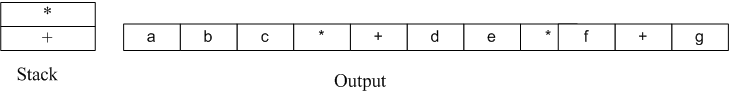15）此时输入数据已经读到末尾，栈中还有两个操作符“*”和" + "，直接弹出并输出。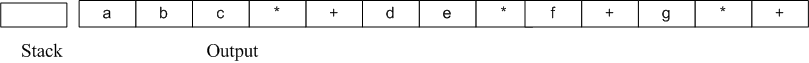至此整个转换过程完成。程序实现代码后续再补充了。

2.3）转换的另一种方法

1)先按照运算符的优先级对中缀表达式加括号，变成( ( a+(b*c) ) + ( ((d*e)+f) *g ) )

2)将运算符移到括号的后面，变成((a(bc)*)+(((de)*f)+g)*)+

3)去掉括号，得到abc*+de*f+g*+

展开全文• 中缀表达式转换为后缀表达式（oj题库） 中缀表达式转换为后缀表达式（oj题库） 题目描述 中缀表达式是一个通用的算术或逻辑公式表示方法，操作符是以中缀形式处于操作数的中间（例：3 + 4），中缀表达式是人们常用的...
• 主要为大家详细介绍了C语言实现中缀表达式转换为后缀表达式，文中示例代码介绍的非常详细，具有一定的参考价值，感兴趣的小伙伴们可以参考一下
• 该程序实现了运算表达式转换为中缀表达式、中缀表达式转换为后缀表达式及后缀表达式求值。该程序已实现加减乘除括号运算符及求余、幂指数的求解
• 栈的应用——表达式求解，内容主要有 中缀表达式转换成后缀表达式以及求解过程，需要可自行下载
• ## 原表达式转换为后缀表达式

万次阅读 多人点赞 2017-08-10 20:42:31
但是要把表达式丢给计算机去处理，它并不能像人一样有逻辑的去判断先处理哪一步，后处理哪一步，它只会严格的按照从左只有执行，因此为了符合计算机运行方式，必须把原表达式转换为对应的后缀表达式才行。...数据结构
• 请设计程序将中缀表达式转换为后缀表达式。 输入格式: 输入在一行中给出不含空格的中缀表达式，可包含+、-、*、\以及左右括号()，表达式不超过20个字符。 输出格式: 在一行中输出转换后的后缀表达式，要求不同...c++ c语言 算法 数据结构
• 转换思路： 1、先对输入的字符串进行处理，分割字符串，划分优先级，将多位数的数字存入结构体看做整体，方便后续处理，为各个运算符划分优先级 2、创建两个栈，一个是结果栈，一个是运算符栈 3、从右至左扫描...
• 中缀表达式转换为后缀表达式 1.创建栈 2.从左向右顺序获取中缀表达式 a.数字直接输出 b.运算符 情况一：遇到左括号直接入栈，遇到右括号将栈中左括号之后入栈的运算符全部弹栈输出，同时左括号出栈但是不输出。...
• ## PTA 表达式转换

千次阅读 2018-06-12 02:52:32
3-1 表达式转换 算术表达式有前缀表示法、中缀表示法和后缀表示法等形式。日常使用的算术表达式是采用中缀表示法，即二元运算符位于两个运算数中间。请设计程序将中缀表达式转换为后缀表达式。 输入格式: 输入在...
• 请设计程序将中缀表达式转换为后缀表达式。 输入格式: 输入在一行中给出不含空格的中缀表达式，可包含+、-、*、\以及左右括号()，表达式不超过20个字符。 输出格式: 在一行中输出转换后的后缀表达式，要求不同对象...
• 7-20表达式转换(25分)算术表达式有前缀表示法、中缀表示法和后缀表示法等形式。日常使用的算术表达式是采用中缀表示法，即二元运算符位于两个运算数中间。请设计程序将中缀表达式转换为后缀表达式。输入格式:输入在...
• 作用 表达式转换属于消极转换组件，换言之是单行运算，来源的列值通过一系列表达式转换（函数转换）后得出的一行输出结果。 注意：多行运算请使用聚合转换组件。 使用 要使用表达式转换进行单行运算，必须要包含以下...
• 表达式转换（25 分） 算术表达式有前缀表示法、中缀表示法和后缀表示法等形式。日常使用的算术表达式是采用中缀表示法，即二元运算符位于两个运算数中间。请设计程序将中缀表达式转换为后缀表达式。 输入...PAT...